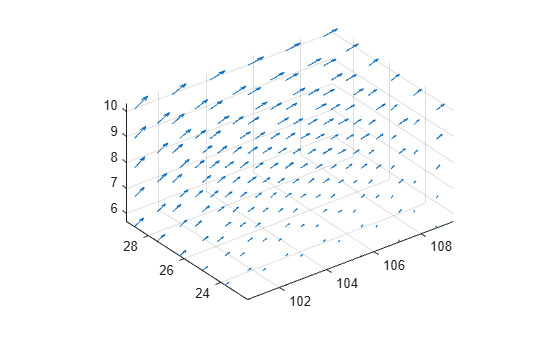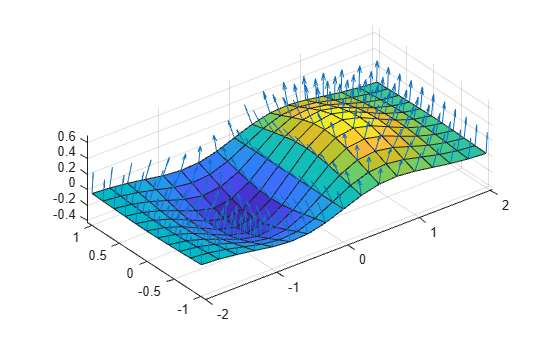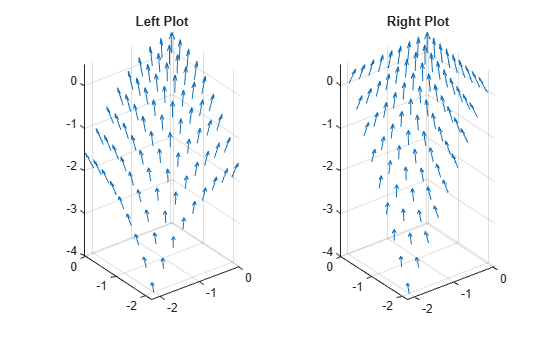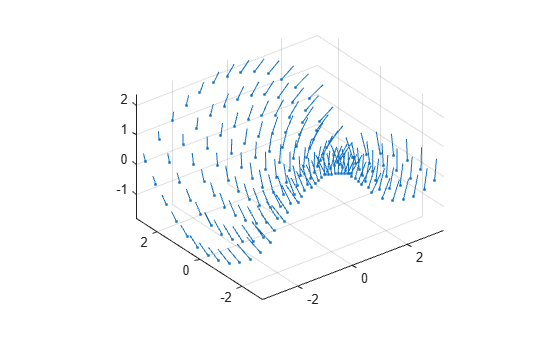quiver3

3-D quiver or vector plot

•Description

example

quiver3(X,Y,Z,U,V,W) plots arrows with directional components U, V, and W at the Cartesian coordinates specified by X, Y, and Z. For example, the first arrow originates from the point X(1), Y(1), and Z(1), extends in the direction of the x-axis according to U(1), extends in the direction of the y-axis according to V(1), and extends in the direction of the z-axis according to W(1). By default, the quiver3 function scales the arrow lengths so that they do not overlap.

quiver3(Z,U,V,W) plots arrows with directional components specified by U, V, and W at equally spaced points along the surface Z.

• If Z is a vector, then the x-coordinates of the arrows range from 1 to the number of elements in Z and the y-coordinates are all 1.

• If Z is a matrix, then the x-coordinates of the arrows range from 1 to the number of columns in Z and the y-coordinates range from 1 to the number of rows in Z.

example

quiver3(___,scale) adjusts the length of arrows:

• When scale is a positive number, the quiver3 function automatically adjusts the lengths of arrows so they do not overlap, then stretches them by a factor of scale. For example, a scale of 2 doubles the length of arrows, and a scale of 0.5 halves the length of arrows.

• When scale is 'off' or 0, such as quiver3(X,Y,Z,U,V,W,'off'), then automatic scaling is disabled.

example

quiver3(___,LineSpec) sets the line style, marker, and color. Markers appear at the points specified by X, Y, and Z. If you specify a marker using LineSpec, then quiver3 does not display arrowheads. To specify a marker and display arrowheads, set the Marker property instead.

quiver3(___,LineSpec,'filled') fills the markers specified by LineSpec.

quiver3(___,Name,Value) specifies quiver properties using one or more name-value pair arguments. For a list of properties, see Quiver Properties. Specify name-value pair arguments after all other input arguments. Name-value pair arguments apply to all of the arrows in the quiver plot.

example

quiver3(ax,___) creates the quiver plot in the axes specified by ax instead of the current axes (gca). The argument ax can precede any of the input argument combinations in the previous syntaxes.

example

q = quiver3(___) returns a Quiver object. This object is useful for controlling the properties of the quiver plot after creating it.

Examples

collapse all

Load sample data that represents air currents over North America. For this example, select a subset of the data.

X = x(5:10,20:25,6:10);
Y = y(5:10,20:25,6:10);
Z = z(5:10,20:25,6:10);
U = u(5:10,20:25,6:10);
V = v(5:10,20:25,6:10);
W = w(5:10,20:25,6:10);

Create a 3-D quiver plot of the subset you selected. The vectors X, Y, and Z represent the location of the base of each arrow, and U, V, and W represent the directional components of each arrow. By default, the quiver3 function shortens the arrows so they do not overlap. Call axis equal to use equal data unit lengths along each axis. This makes the arrows point in the correct direction.

quiver3(X,Y,Z,U,V,W)
axis equalBy default, the quiver3 function shortens arrows so they do not overlap. To disable automatic scaling so that arrow lengths are determined entirely by U, V, and W, set the scale argument to 0.

For example, first return the x-, y-, and z-coordinates of a unit sphere with 10-by-10 faces. Calculate the directional components of its surface normals using the surfnorm function. Then, create a 3-D quiver plot with no automatic scaling.

[X,Y,Z] = sphere(10);
[U,V,W] = surfnorm(X,Y,Z);
quiver3(X,Y,Z,U,V,W,0)
axis equalFor comparison, create the plot with automatic scaling. Note that the arrows are shorter and do not overlap.

figure
quiver3(X,Y,Z,U,V,W)
axis equalPlot vectors that are normal to the surface defined by the function $z=x{e}^{-{x}^{2}-{y}^{2}}$. Use the quiver3 function to plot the vectors and the surf function to plot the surface.

First, create a grid of x- and y-values that are equally spaced. Use them to calculate z. Then, find the normal vectors.

[X,Y] = meshgrid(-2:0.25:2,-1:0.2:1);
Z = X.*exp(-X.^2 - Y.^2);
[U,V,W] = surfnorm(X,Y,Z);

Display the vectors as a 3-D quiver plot. Then, display the surface in the same axes. Adjust the display so that the vectors appear normal to the surface by calling axis equal.

quiver3(X,Y,Z,U,V,W)
hold on
surf(X,Y,Z)
axis equalCreate a 3-D quiver plot and specify a color for the arrows.

For example, first return the x-, y-, and z- coordinates of a surface. Calculate the directional components of its surface normals using the surfnorm function.

[X,Y] = meshgrid(-pi/2:pi/8:pi/2,-pi/2:pi/8:pi/2);
Z = sin(X) + cos(Y);
[U,V,W] = surfnorm(Z);

Then, create a 3-D quiver plot with red arrows.

quiver3(X,Y,Z,U,V,W,'r')
axis equalStarting in R2019b, you can display a tiling of plots using the tiledlayout and nexttile functions. Call the tiledlayout function to create a 1-by-2 tiled chart layout. Call the nexttile function to create an axes object and return the object as ax1. Create the left plot by passing ax1 to the quiver3 function. Add a title to the plot by passing the axes to the title function. Repeat the process to create the right plot.

[X,Y] = meshgrid(-2:0.25:0,-2:0.25:0);
Z1 = -0.5*(X.^2 + Y.^2);
[U1,V1,W1] = surfnorm(Z1);
Z2 = -X.*Y;
[U2,V2,W2] = surfnorm(Z2);

tiledlayout(1,2)

% Left plot
ax1 = nexttile;
quiver3(ax1,X,Y,Z1,U1,V1,W1)
axis equal
title(ax1,'Left Plot')

% Right plot
ax2 = nexttile;
quiver3(ax2,X,Y,Z2,U2,V2,W2)
axis equal
title(ax2,'Right Plot')Create a 3-D quiver plot and return the quiver object. Then, remove the arrowheads and add dot markers at the base of each arrow.

[X,Y] = meshgrid(-3:0.5:3,-3:0.5:3);
Z = 0.2*(Y.^2 - X.^2);
[U,V,W] = surfnorm(Z);

q = quiver3(X,Y,Z,U,V,W);
axis equal
q.Marker = '.';Input Arguments

collapse all

x-coordinates of the bases of arrows, specified as a scalar, a vector, or a matrix.

If X and Y are vectors and Z, U, V, and W are matrices, then quiver3 expands X and Y into matrices. In this case, size(Z), size(U), size(V), and size(W) must equal [length(Y) length(X)]. For more information about expanding vectors into matrices, see meshgrid.

If X and Y are matrices, then X, Y, Z, U, V, and W must be the same size.

y-coordinates of the bases of arrows, specified as a scalar, a vector, or a matrix.

If X and Y are vectors and Z, U, V, and W are matrices, then quiver3 expands X and Y into matrices. In this case, size(Z), size(U), size(V), and size(W) must equal [length(Y) length(X)]. For more information about expanding vectors into matrices, see meshgrid.

If X and Y are matrices, then X, Y, Z, U, V, and W must be the same size.

z-coordinates of the bases of arrows, specified as a scalar, a vector, or a matrix.

If X and Y are vectors and Z is a matrix, then size(Z) must equal [length(Y) length(X)].

If X and Y are matrices, then X, Y, Z, U, V, and W must be the same size.

x-components of arrows, specified as a scalar, vector, or matrix.

If X and Y are vectors and U is a matrix, then size(U) must equal [length(Y) length(X)].

If X and Y are matrices, then X, Y, Z, U, V, and W must be the same size.

y-components of arrows, specified as a scalar, vector, or matrix.

If X and Y are vectors and V is a matrix, then size(V) must equal [length(Y) length(X)].

If X and Y are matrices, then X, Y, Z, U, V, and W must be the same size.

z-components of arrows, specified as a scalar, vector, or matrix.

If X and Y are vectors and W is a matrix, then size(W) must equal [length(Y) length(X)].

If X and Y are matrices, then X, Y, Z, U, V, and W must be the same size.

Line style, marker, and color, specified as a character vector or string containing symbols. The symbols can appear in any order. You do not need to specify all three characteristics (line style, marker, and color).

If you specify a marker using LineSpec, then quiver3 does not display arrowheads. To specify a marker and display arrowheads, set the Marker property instead.

Example: '--or' is a red dashed line with circle markers

Line StyleDescriptionResulting Line
'-'Solid line'--'Dashed line':'Dotted line'-.'Dash-dotted lineMarkerDescriptionResulting Marker
'o'Circle'+'Plus sign'*'Asterisk'.'Point'x'Cross'_'Horizontal line'|'Vertical line's'Square'd'Diamond'^'Upward-pointing triangle'v'Downward-pointing triangle'>'Right-pointing triangle'<'Left-pointing triangle'p'Pentagram'h'HexagramColor NameShort NameRGB TripletAppearance
'red''r'[1 0 0]'green''g'[0 1 0]'blue''b'[0 0 1]'cyan' 'c'[0 1 1]'magenta''m'[1 0 1]'yellow''y'[1 1 0]'black''k'[0 0 0]'white''w'[1 1 1]Arrow scaling factor, specified as a positive number or 'off'. By default, the quiver3 function automatically scales the arrows so they do not overlap. The quiver3 function applies the scaling factor after it automatically scales the arrows.

Specifying scale is the same as setting the AutoScaleFactor property of the quiver object. For example, specifying scale as 2 doubles the length of the arrows. Specifying scale as 0.5 halves the length of the arrows.

To disable automatic scaling, specify scale as 'off' or 0. When you specify either of these values, the AutoScale property of the quiver object is set to 'off' and the length of the arrow is determined entirely by U, V, and W.

Target axes, specified as an Axes object. If you do not specify the axes, then the quiver3 function uses the current axes.

Name-Value Arguments

Specify optional comma-separated pairs of Name,Value arguments. Name is the argument name and Value is the corresponding value. Name must appear inside quotes. You can specify several name and value pair arguments in any order as Name1,Value1,...,NameN,ValueN.

Example: 'Color','r','LineWidth','1

Note

The properties listed here are only a subset. For a complete list, see Quiver Properties.

Width of arrow stem and head, specified as a scalar numeric value greater than zero in point units. One point equals 1/72 inch. The default value is 0.5 point.

Example: 0.75

Arrowhead display, specified as 'on' or 'off', or as numeric or logical 1 (true) or 0 (false). A value of 'on' is equivalent to true, and 'off' is equivalent to false. Thus, you can use the value of this property as a logical value. The value is stored as an on/off logical value of type matlab.lang.OnOffSwitchState.

• 'on' — Display the vectors with arrowheads.

• 'off' — Display the vectors without arrowheads.

Automatic scaling of arrow length, specified as 'on' or 'off', or as numeric or logical 1 (true) or 0 (false). A value of 'on' is equivalent to true, and 'off' is equivalent to false. Thus, you can use the value of this property as a logical value. The value is stored as an on/off logical value of type matlab.lang.OnOffSwitchState.

• 'on' — Scale the arrow length to fit within the grid-defined coordinate data and scale arrows so that they do not overlap. The quiver or quiver3 function then applies the AutoScaleFactor to the arrow length.

• 'off' — Do not scale the arrow lengths.

Scale factor, specified as a scalar. A value of 2 doubles the length of the arrows. A value of 0.5 halves the length of the arrows.

This property has an effect only if the AutoScale property is set to 'on'.

Example: 2

Tips

To create a 3-D quiver plot using cylindrical or spherical coordinates, first convert them to Cartesian coordinates using the pol2cart or sph2cart function.